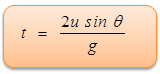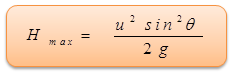×#### Thank you for registering.

One of our academic counsellors will contact you within 1 working day.

Click to Chat

1800-1023-196

+91-120-4616500

CART 0

• 0

MY CART (5)

Use Coupon: CART20 and get 20% off on all online Study Material

ITEM
DETAILS
MRP
DISCOUNT
FINAL PRICE
Total Price: Rs.

There are no items in this cart.
Continue Shopping

Pls. explain equation of trajectory motion or give a suitable link.


2 years ago

							 A body projected into the space and is no longer being propelled by fuel is called a projectile.To analyze the projectile motion we use the following concept "Resolution of two dimensional motion into two one dimension motion" as discussed earlier. Hence it is easier to analyze the motion of projectile as composed of two simultaneous rectilinear motions which are independent of each other:(a) Along the vertical y-axis with a uniform downward acceleration 'g' and(b) Along the horizontal x-axis with a uniform velocity forward.Consider a particle projected with an initial velocity u at an angle θ with the horizontal x-axis as shown in figure shown below. Velocity and accelerations can be resolved into two components:Velocity along x-axis = ux = u cos θAcceleration along x-axis ax = 0Velocity along y-axis = uy = u sin θAcceleration along y-axis ay = -gHere we use different equation of motions of one dimension derived earlier to get the different parameters.                           …... (a)            …... (b)         v2 = v02 – 2g (y-y0)                      …... (c)  Total Time of FlightWhen body returns to the same horizontal level, the resultant displacement in vertical y-direction is zero. Use equation b.Therefore, 0 = (u sin θ) t - (½)gt2,As t cannot equal to zero, then, total time of flight,Horizontal RangeHorizontal Range (OA=X)   = Horizontal velocity × Time of flight= u cos θ × 2 u sin θ/gSo horizontal range,Maximum HeightAt the highest point of the trajectory, vertical component of velocity is zero.Therefore, 0 = (u sin θ)2 - 2g HmaxSo, maximum height would be,Equation of TrajectoryAssuming the point of projection as the origin of co-ordinates and horizontal direction as the x-axis and vertical direction as the y-axis. Let P (x, y) be the position of the particle at instant after t second.Then x = u cos θ.t and y = u sin θ.t - 1/2 gt2Eliminating 't' form the above equations, we get,y = x tan θ - (gx2/2u2cos2θ)This is the equation of trajectory which is a parabola (y = ax + bx2).

2 years ago
Think You Can Provide A Better Answer ?

## Other Related Questions on Mechanics

View all Questions »### Course Features

• 101 Video Lectures
• Revision Notes
• Previous Year Papers
• Mind Map
• Study Planner
• NCERT Solutions
• Discussion Forum
• Test paper with Video Solution### Course Features

• 110 Video Lectures
• Revision Notes
• Test paper with Video Solution
• Mind Map
• Study Planner
• NCERT Solutions
• Discussion Forum
• Previous Year Exam Questions